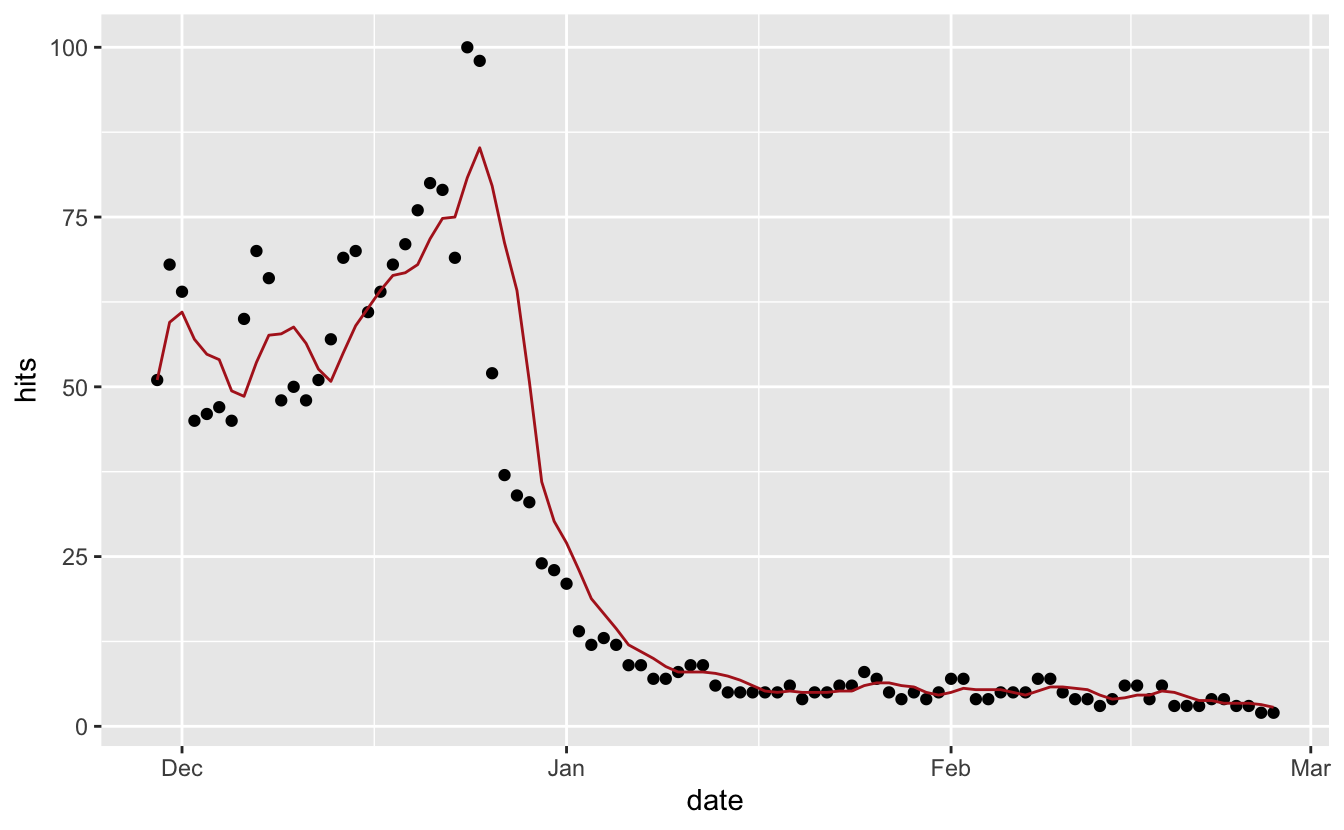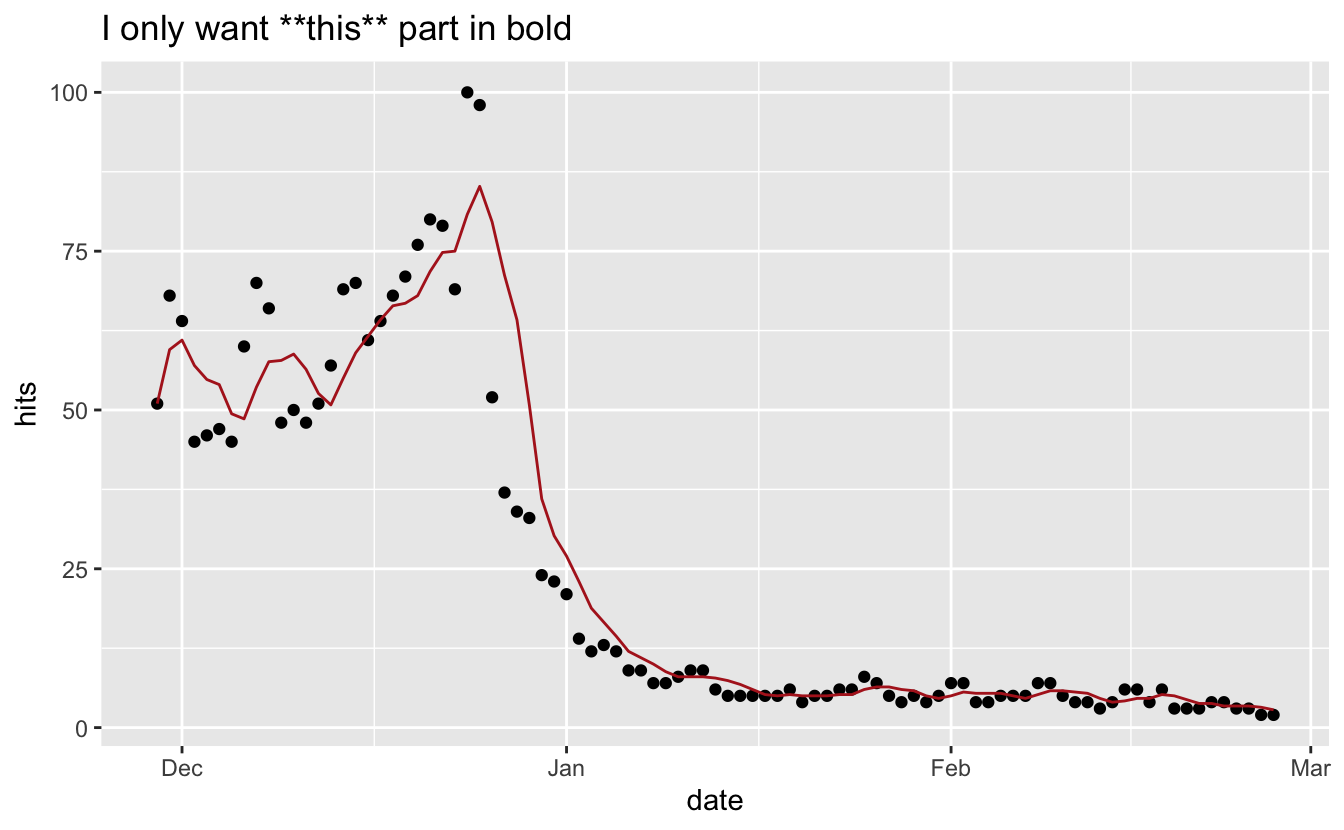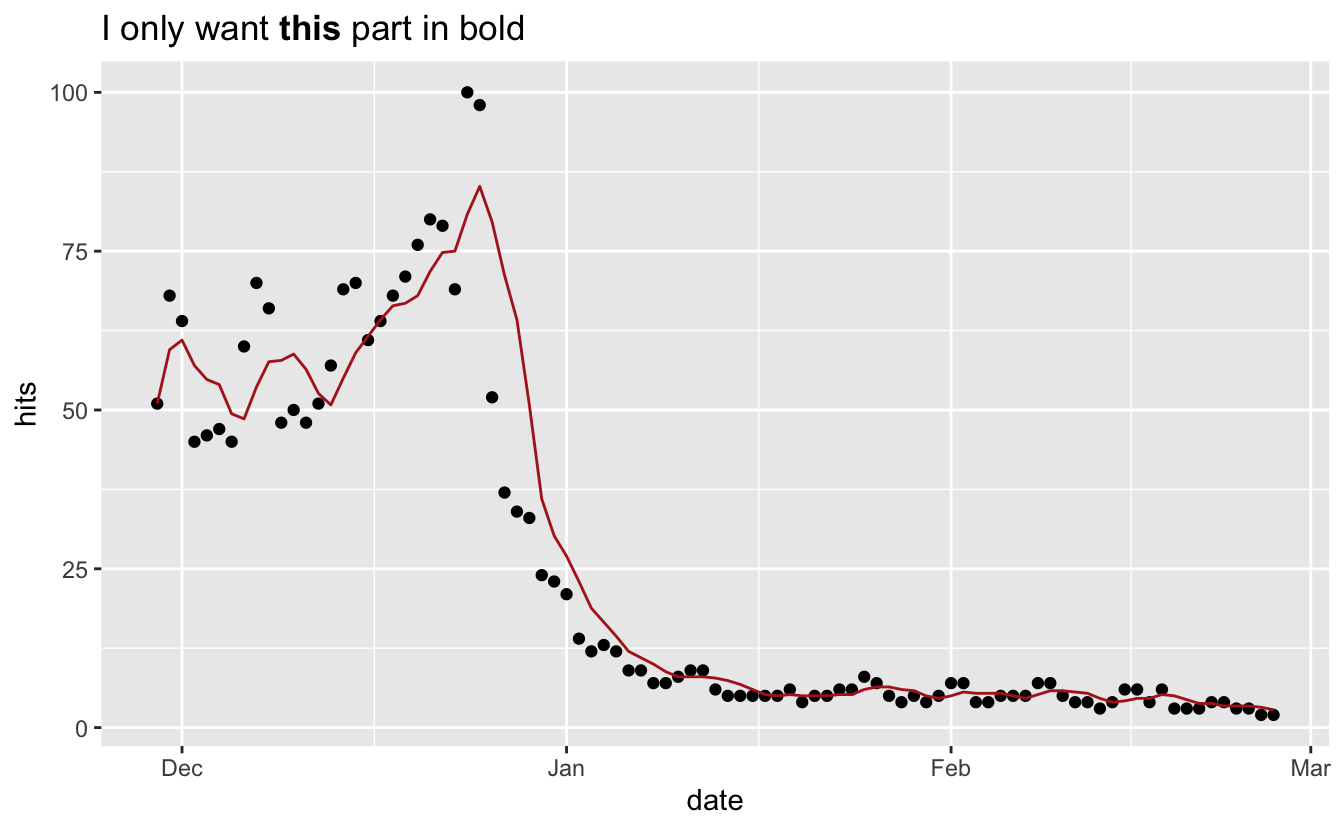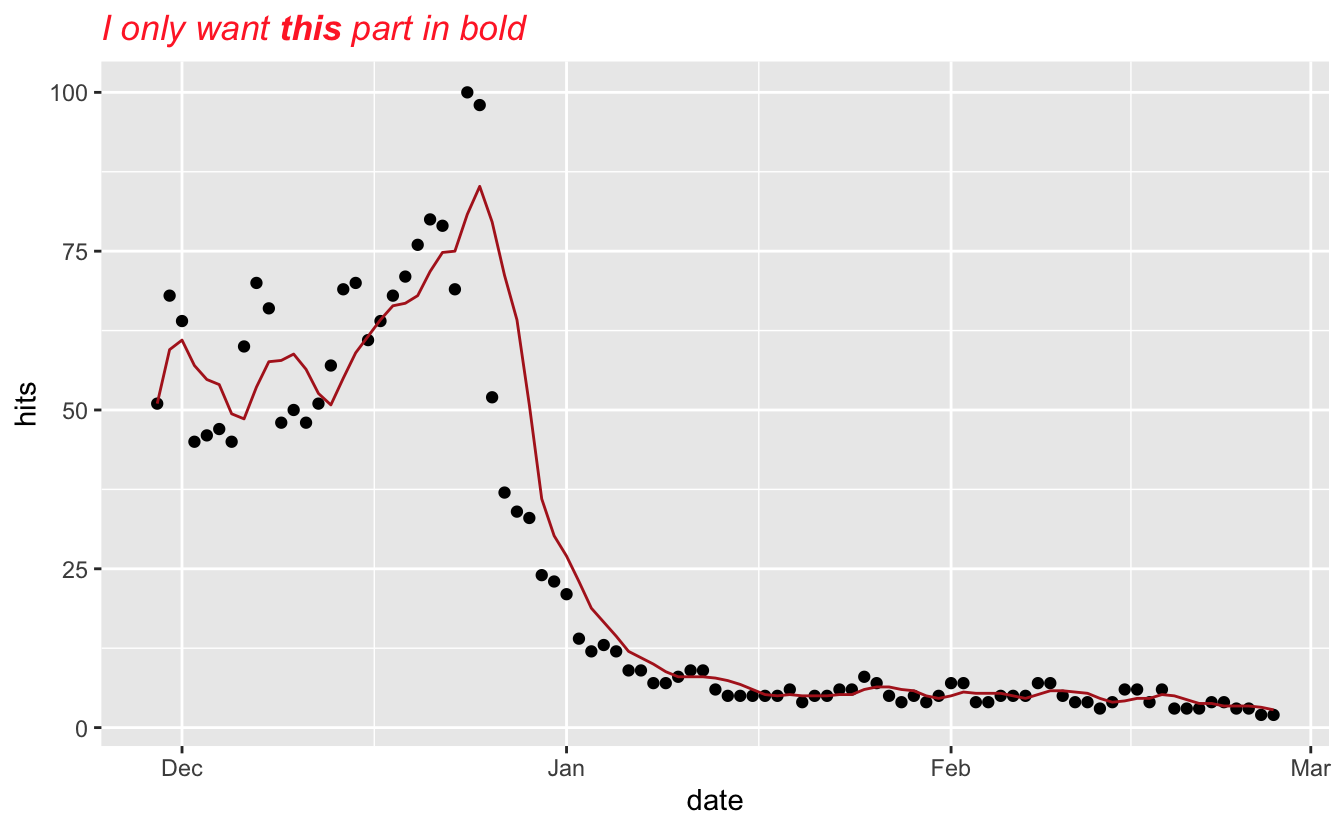# Day 19: ggtext

Welcome back for the 19th day of the #packagecalendar, today we will be taking a look at the ggtext package by Claus Wilke. The ggtext package provides improved text rendering support for ggplot2.

The package is not yet available from CRAN but can be downloaded with

``remotes::install_github("clauswilke/ggtext")``

For the following example, we will use the “Last Christmas” data from earlier posts.

``````# remotes::install_github("PMassicotte/gtrendsR")
library(gtrendsR)
last_christmas <- gtrends("Last Christmas", time = "today 3-m")\$interest_over_time``````

And we will be building off the chart we created back in day 11

``````library(ggplot2)
library(slide)
library(dplyr)
last_christmas %>%
mutate(hits_ma5 = slide_dbl(hits, ~mean(.x), .before = 4)) %>%
ggplot(aes(date, hits)) +
geom_point() +
geom_line(aes(y = hits_ma5), color = "firebrick")``````ggtext introduces a new theme element, `element_markdown()`, which behaves similarly to `element_text()` but renders the text as markdown/HTML. Suppose you want to selectively bold part of our title. Using markdown we would put the text in double stars.

``````last_christmas %>%
mutate(hits_ma5 = slide_dbl(hits, ~mean(.x), .before = 4)) %>%
ggplot(aes(date, hits)) +
geom_point() +
geom_line(aes(y = hits_ma5), color = "firebrick") +
labs(title = "I only want **this** part in bold")``````But using normal ggplot2 doesn’t allow you to do that. There is where ggtext comes in, we use the `theme()` function to specify the plot title to be rendered using markdown.

``````library(ggtext)
last_christmas %>%
mutate(hits_ma5 = slide_dbl(hits, ~mean(.x), .before = 4)) %>%
ggplot(aes(date, hits)) +
geom_point() +
geom_line(aes(y = hits_ma5), color = "firebrick") +
labs(title = "I only want **this** part in bold") +
theme(plot.title = element_markdown())``````Additionally, you can also use standard HTML syntax to modify your text

``````last_christmas %>%
mutate(hits_ma5 = slide_dbl(hits, ~mean(.x), .before = 4)) %>%
ggplot(aes(date, hits)) +
geom_point() +
geom_line(aes(y = hits_ma5), color = "firebrick") +
labs(title =  "<i style='color:#FF3030'>I only want **this** part in bold</i>") +
theme(plot.title = element_markdown())``````